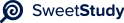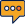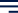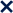•01 / 2100%Median Housing Price Prediction Model for D.M. Pan Real Estate Company
Ashley Allen
Southern New Hampshire University
Regression Equation
Equation of regression: y = 146.52x - 9561
Determine r
The relationship between variables depends on [rvalue. ]'s The square root, or [r] value, is 0.988
since the [r2] value is 0.713. Strong correlation between the variables is indicated by a [r] value
of between 0.8 and 1. If [r] is positive, then the slope is positive and moves in the right-up
direction. This demonstrates that as one variable rises, the other one rises as well. If Y rises,
then X must also increase, or vice versa. In other words, the listing price of the home rises as
the home's square footage does.
Examine the Slope and Intercepts
y = 146.52x - 95611 is the formula for the slope intercept. Based on two points on the line and
the Y intercept, we calculated the slope. The slope of the linear regression line may also be
determined by taking two points on the line. 95611 is the Y-intercept. b0 is 95611. The slope
(increase over run), which is given by b1, is 146.52. I don't think this slope intercept is correct; it
seems like it should be closer to 50,000.
R-squared Coefficient
The proportional connection between the independent and dependent variables in a regression
model is represented by the r2 value. It details the degree to which one variable affects the
other. Since the inputs can account for roughly 3/4 of the observed variance, the r2 value is
0.713.
Conclusions
As a result, we are aware that the square footage and listing price of the homes are directly
related. The listing price and increased square footage are directly, strongly, and favorably
correlated (causing it to increase as well). The national average delivers greater prices per
square foot than the random picks I made, according to this connection. Different random
samples could have different outcomes. I could determine the deviation more precisely if I
entered the national average into a regression model. Finding the ideal listing price for a home
may be done by knowing the slope and r value. We would be in a better position to decide what
the house should be listed at in relation to the area's average if there was a chart that showed
the regression line and slope for the average house listing price in the nearby region.The listing price of a home should rise by around \$8,300 for every 100 square feet. Given that
the median price per square foot is \$83, the price would rise by about \$8300 for 100 square
feet. We can calculate the appropriate listing price for a 1200 square foot home using the
regression equation, which is \$80,213. The graph works well as an illustration for homes with a
square footage of 1500 to 2000.